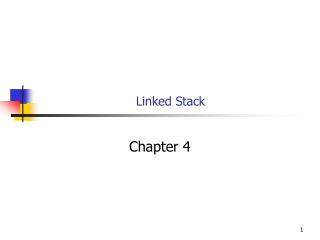DownloadDownload PresentationDownload Presentation- - - - - - - - - - - - - - - - - - - - - - - - - - - E N D - - - - - - - - - - - - - - - - - - - - - - - - - - -
##### Presentation Transcript

2. Linked Stack • We can implement a stack as a linked list. • Same operations. • No fixed maximum size. • Stack can grow indefinitely • Subject only to the amount of memory available.

3. Linked Stack • Rather than implementing a linked stack from scratch we will use an existing Link class and create an adapter. • A wrapper that provides a different interface for an existing class. • Using a linked list to implement a stack is especially simple. • The operations provided by the Stack ADT are a subset of those provided by the List ADT.

4. Getting Started • Create a new empty project • Linked_Stack • Copy stack.h and stack_test.cpp from last class into the new Linked_Stack project • http://www.cse.usf.edu/~turnerr/Data_Structures/Downloads/2011_02_07_Stacks/ • Add to project. • Project > Add Existing Item

5. Getting Started • Download final List Template project: • http://www.cse.usf.edu/~turnerr/Data_Structures/Downloads/2011_02_02_List_of_Objects/Completed_and_Corrected_Version/ • Extract files. • Copy DLList.h into new project. • Add file to project. • Note memory leak in destructor. • Modify stack.h as shown on following slides. We will use a List to hold the stack data.

6. Stack.h #pragma once #include <iostream> #include <cassert> #include "DLList.h" template <class T> class Stack { public: Stack(int capacity = 1000); ~Stack(); bool IsEmpty() const {return data->isEmpty();}; bool IsFull() const {return false;}; // Add a value to the top of the stack. void Push(const T& value); // Remove and return value at top of stack. T Pop(); // Retrieve value at top of stack without removing it. T Top() const; // Display stack contents. void Display(std::ostream& out) const;

7. Stack.h private: DLList<T>* data; //int size; //int top; // Index of element at top of stack // // -1 when stack is empty };

8. Constructor and Destructor template <class T> Stack<T>::Stack(int capacity)Delete initialization list { data = new DLList<T>(); assert (data != 0); } template <class T> Stack<T>::~Stack() { delete data;Remove [] }

9. Push() template <class T> void Stack<T>::Push(const T& value) { data->addToHead(value); }

10. Pop() template <class T> T Stack<T>::Pop() { if (this->IsEmpty()) { throw "Pop called for empty stack"; } return data->deleteFromHead(); }

11. Top() template <class T> T Stack<T>::Top() const { if (this->IsEmpty()) { throw "Top of empty stack requested"; } T temp = data->deleteFromHead(); data->addToHead(temp); return temp; }

12. Display() template <class T> void Stack<T>::Display(std::ostream& out) const { data->printAll(); }

13. stack_test • Our test should work unchanged • Except: We can't force overflow now. • Comment out the section that tests stack overflow. • Lines 28 – 41 of stack_test.cpp • Build

14. A Warning The isEmpty method in the List template should have been declared as bool. Make that change.

15. Program Running

16. Try it on Linux

17. Try it on Linux • The Visual Studio compiler is more forgiving! • Add using namespace std; to printAll.

18. OK This Time

19. An Application • Adding arbitrarily large numbers. • Unlike pencil and paper arithmetic, computer arithmetic typically has a maximum size for numbers. • We can use stacks to implement addition for arbitrarily large numbers. • Drozdek, page 141

20. Modify printAll() • Modify DLList::printAll() to output all items on the same line. cout << p->info << " ";

21. Adding Arbitrarily Large Integers int main() { Stack<int> lhs; Stack<int> rhs; Stack<int> result; get_integer(lhs); get_integer(rhs); cout << "\nLHS = "; lhs.Display(cout); cout << "\nRHS = "; rhs.Display(cout); cin.get(); cin.get(); }

22. get_integer() void get_integer(Stack<int>& stack) { cout << "Enter an integer: "; char c = cin.get(); while (c != '\n') { assert (isdigit(c)); stack.Push(c - '0'); c = cin.get(); } }

23. Program in Action

24. Program in Action

25. Add to main() add(lhs, rhs, result); cout << "\nSum = "; result.Display(cout); cout << endl;

26. Function Add() void add(Stack<int>& lhs, Stack<int>& rhs, Stack<int>& result) { int carry = 0; while (!lhs.IsEmpty() || !rhs.IsEmpty() || carry > 0) { int next_lhs_digit = 0; int next_rhs_digit = 0; if (!lhs.IsEmpty()) { next_lhs_digit = lhs.Pop(); } if (!rhs.IsEmpty()) { next_rhs_digit = rhs.Pop(); }

27. Function Add() int next_sum_digit = next_lhs_digit + next_rhs_digit + carry; if (next_sum_digit > 9) { carry = 1; next_sum_digit -= 10; } else { carry = 0; } result.Push(next_sum_digit); } }

28. A Small Example

29. A Big Example

30. Another Big Example